Publicité

# Chap 2 Arrays and Structures.ppt

9 Nov 2022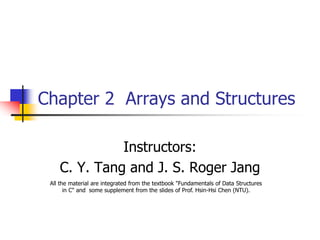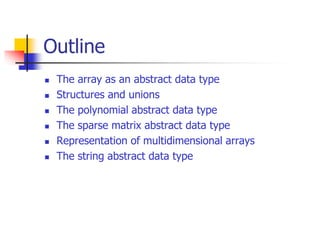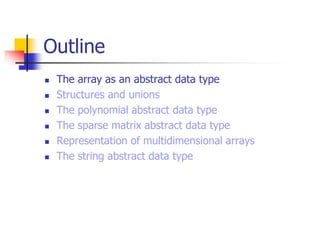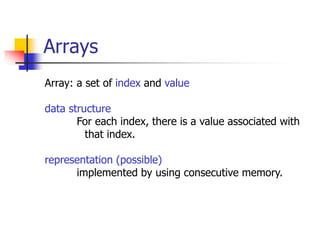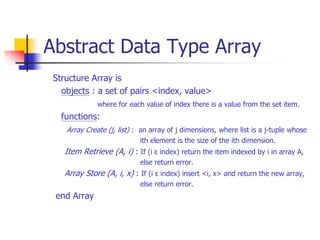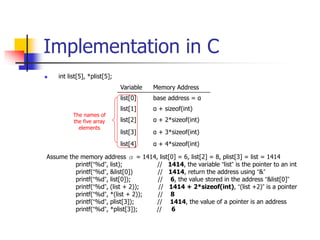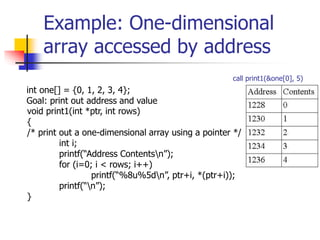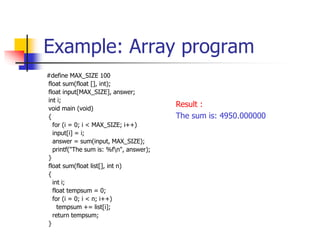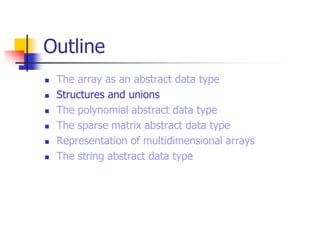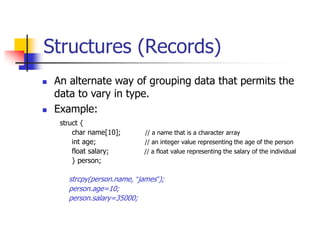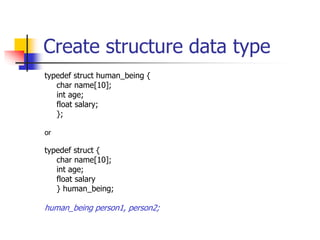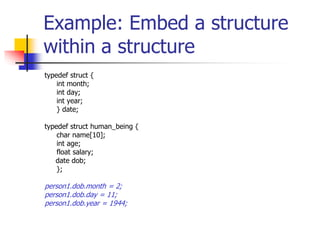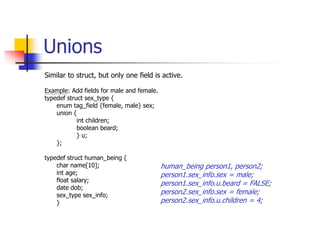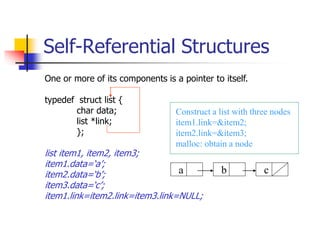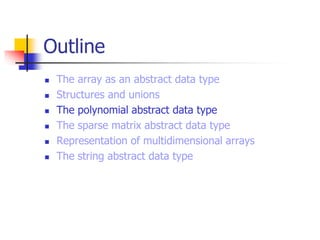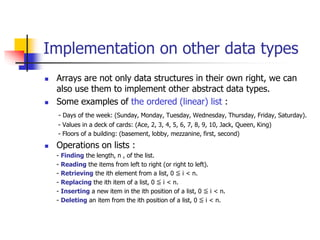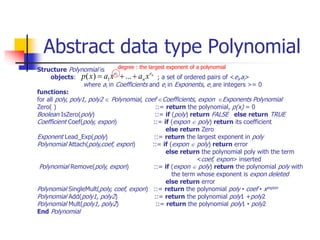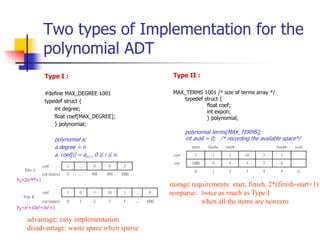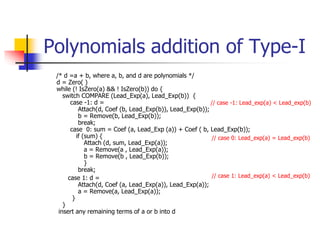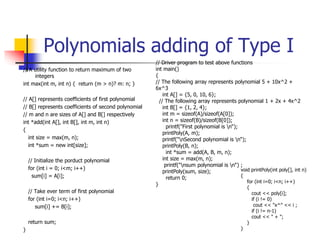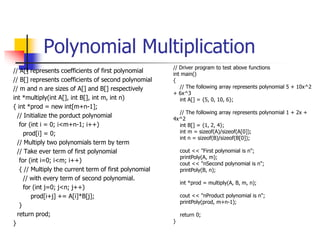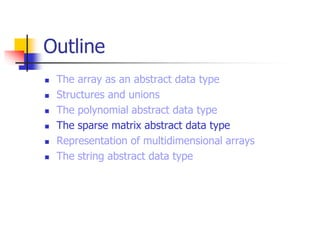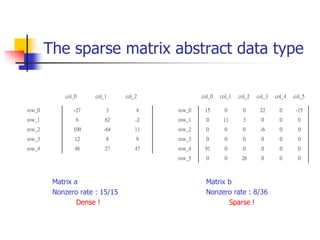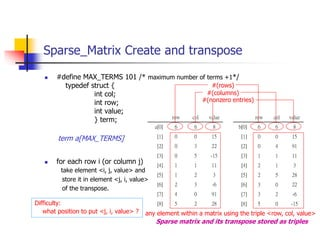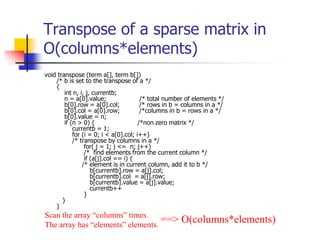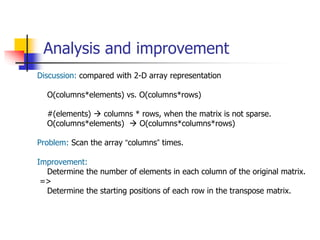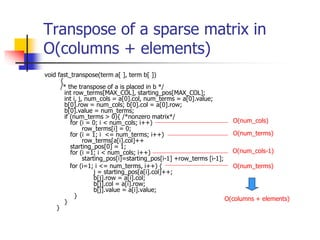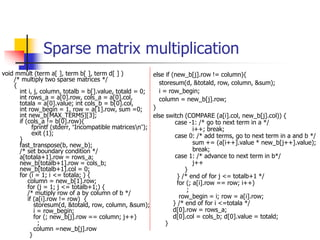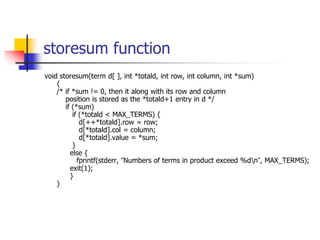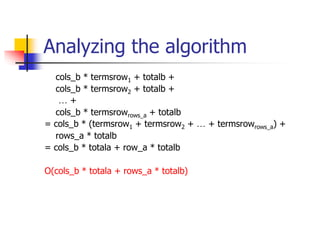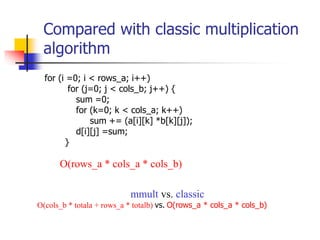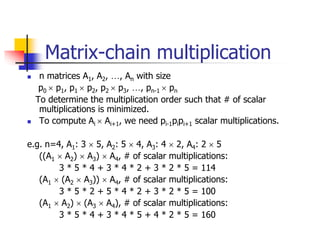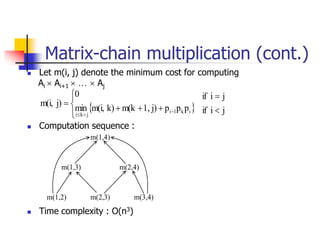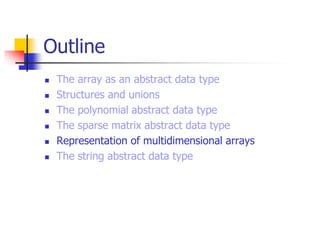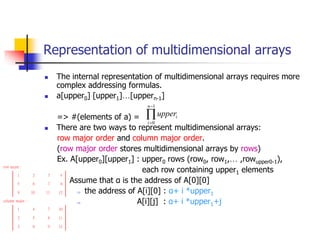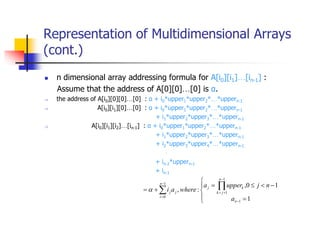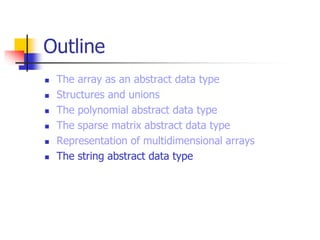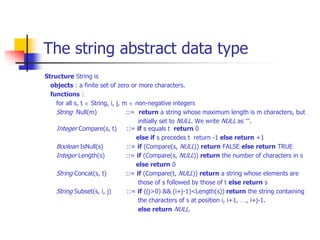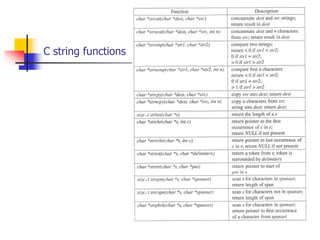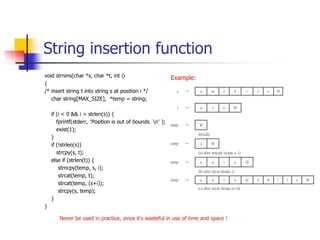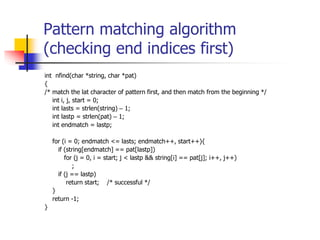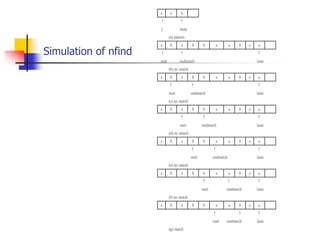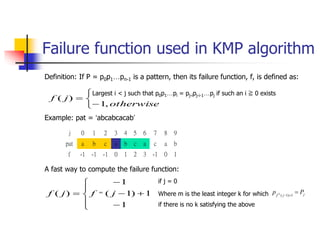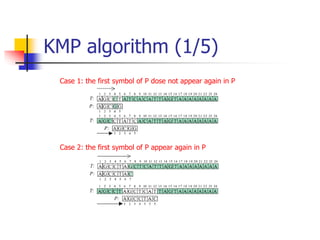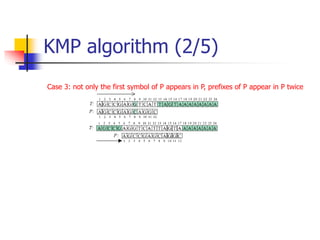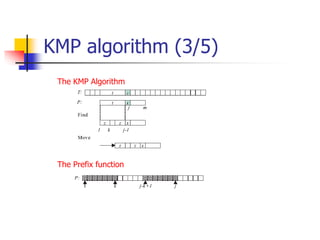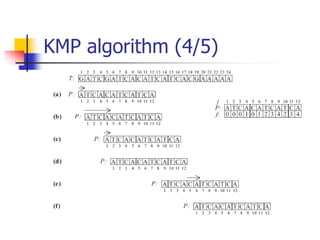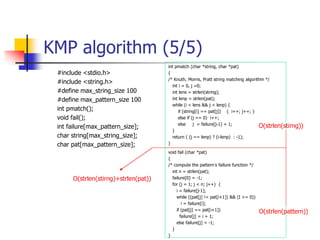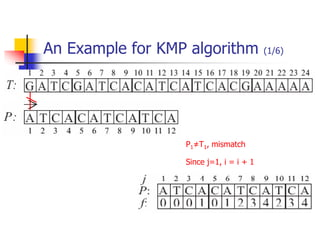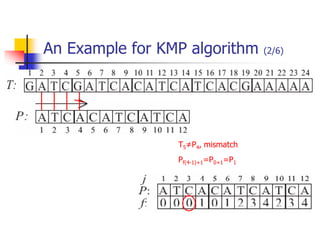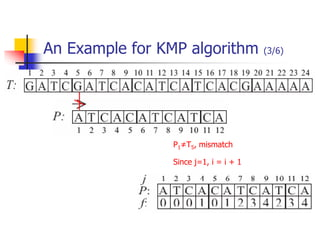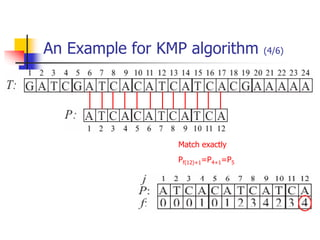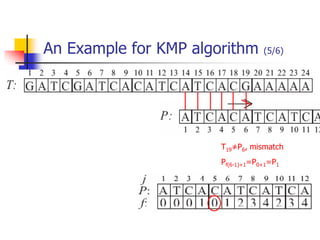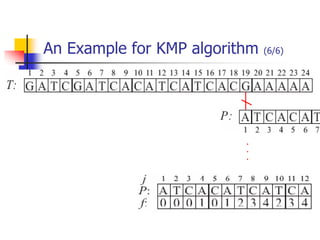1 sur 55
Publicité

### Chap 2 Arrays and Structures.ppt

1. Chapter 2 Arrays and Structures Instructors: C. Y. Tang and J. S. Roger Jang All the material are integrated from the textbook "Fundamentals of Data Structures in C" and some supplement from the slides of Prof. Hsin-Hsi Chen (NTU).
2. Outline  The array as an abstract data type  Structures and unions  The polynomial abstract data type  The sparse matrix abstract data type  Representation of multidimensional arrays  The string abstract data type
3. Outline  The array as an abstract data type  Structures and unions  The polynomial abstract data type  The sparse matrix abstract data type  Representation of multidimensional arrays  The string abstract data type
4. Arrays Array: a set of index and value data structure For each index, there is a value associated with that index. representation (possible) implemented by using consecutive memory.
5. Abstract Data Type Array Structure Array is objects : a set of pairs <index, value> where for each value of index there is a value from the set item. functions: Array Create (j, list) : an array of j dimensions, where list is a j-tuple whose ith element is the size of the ith dimension. Item Retrieve (A, i) : If (i ε index) return the item indexed by i in array A, else return error. Array Store (A, i, x) : If (i ε index) insert <i, x> and return the new array, else return error. end Array
6. Implementation in C  int list, *plist; Variable Memory Address list base address = α list α + sizeof(int) list α + 2*sizeof(int) list α + 3*sizeof(int) list α + 4*sizeof(int) The names of the five array elements Assume the memory address α = 1414, list = 6, list = 8, plist = list = 1414 printf(“%d”, list); // 1414, the variable “list” is the pointer to an int printf(“%d”, &list) // 1414, return the address using “&” printf(“%d”, list); // 6, the value stored in the address “&list” printf(“%d”, (list + 2)); // 1414 + 2*sizeof(int), “(list +2)” is a pointer printf(“%d”, *(list + 2)); // 8 printf(“%d”, plist); // 1414, the value of a pointer is an address printf(“%d”, *plist); // 6
7. Example: One-dimensional array accessed by address call print1(&one, 5) void print1(int *ptr, int rows) { /* print out a one-dimensional array using a pointer */ int i; printf(“Address Contentsn”); for (i=0; i < rows; i++) printf(“%8u%5dn”, ptr+i, *(ptr+i)); printf(“n”); } int one[] = {0, 1, 2, 3, 4}; Goal: print out address and value
8. Example: Array program #define MAX_SIZE 100 float sum(float [], int); float input[MAX_SIZE], answer; int i; void main (void) { for (i = 0; i < MAX_SIZE; i++) input[i] = i; answer = sum(input, MAX_SIZE); printf("The sum is: %fn", answer); } float sum(float list[], int n) { int i; float tempsum = 0; for (i = 0; i < n; i++) tempsum += list[i]; return tempsum; } Result : The sum is: 4950.000000
9. Outline  The array as an abstract data type  Structures and unions  The polynomial abstract data type  The sparse matrix abstract data type  Representation of multidimensional arrays  The string abstract data type
10. Structures (Records) struct { char name; // a name that is a character array int age; // an integer value representing the age of the person float salary; // a float value representing the salary of the individual } person; strcpy(person.name, “james”); person.age=10; person.salary=35000;  An alternate way of grouping data that permits the data to vary in type.  Example:
11. Create structure data type typedef struct human_being { char name; int age; float salary; }; or typedef struct { char name; int age; float salary } human_being; human_being person1, person2;
12. Example: Embed a structure within a structure typedef struct { int month; int day; int year; } date; typedef struct human_being { char name; int age; float salary; date dob; }; person1.dob.month = 2; person1.dob.day = 11; person1.dob.year = 1944;
13. Unions Similar to struct, but only one field is active. Example: Add fields for male and female. typedef struct sex_type { enum tag_field {female, male} sex; union { int children; boolean beard; } u; }; typedef struct human_being { char name; int age; float salary; date dob; sex_type sex_info; } human_being person1, person2; person1.sex_info.sex = male; person1.sex_info.u.beard = FALSE; person2.sex_info.sex = female; person2.sex_info.u.children = 4;
14. Self-Referential Structures One or more of its components is a pointer to itself. typedef struct list { char data; list *link; }; list item1, item2, item3; item1.data=‘a’; item2.data=‘b’; item3.data=‘c’; item1.link=item2.link=item3.link=NULL; a b c Construct a list with three nodes item1.link=&item2; item2.link=&item3; malloc: obtain a node
15. Outline  The array as an abstract data type  Structures and unions  The polynomial abstract data type  The sparse matrix abstract data type  Representation of multidimensional arrays  The string abstract data type
16. Implementation on other data types  Arrays are not only data structures in their own right, we can also use them to implement other abstract data types.  Some examples of the ordered (linear) list : - Days of the week: (Sunday, Monday, Tuesday, Wednesday, Thursday, Friday, Saturday). - Values in a deck of cards: (Ace, 2, 3, 4, 5, 6, 7, 8, 9, 10, Jack, Queen, King) - Floors of a building: (basement, lobby, mezzanine, first, second)  Operations on lists : - Finding the length, n , of the list. - Reading the items from left to right (or right to left). - Retrieving the ith element from a list, 0 ≦ i < n. - Replacing the ith item of a list, 0 ≦ i < n. - Inserting a new item in the ith position of a list, 0 ≦ i < n. - Deleting an item from the ith position of a list, 0 ≦ i < n.
17. Abstract data type Polynomial Structure Polynomial is objects: ; a set of ordered pairs of <ei,ai> where ai in Coefficients and ei in Exponents, ei are integers >= 0 functions: for all poly, poly1, poly2  Polynomial, coef Coefficients, expon Exponents Polynomial Zero( ) ::= return the polynomial, p(x) = 0 Boolean IsZero(poly) ::= if (poly) return FALSE else return TRUE Coefficient Coef(poly, expon) ::= if (expon  poly) return its coefficient else return Zero Exponent Lead_Exp(poly) ::= return the largest exponent in poly Polynomial Attach(poly,coef, expon) ::= if (expon  poly) return error else return the polynomial poly with the term <coef, expon> inserted Polynomial Remove(poly, expon) ::= if (expon  poly) return the polynomial poly with the term whose exponent is expon deleted else return error Polynomial SingleMult(poly, coef, expon) ::= return the polynomial poly • coef • xexpon Polynomial Add(poly1, poly2) ::= return the polynomial poly1 +poly2 Polynomial Mult(poly1, poly2) ::= return the polynomial poly1 • poly2 End Polynomial n e n e x a x a x p    ... ) ( 1 1 degree : the largest exponent of a polynomial
18. Two types of Implementation for the polynomial ADT Type I : #define MAX_DEGREE 1001 typedef struct { int degree; float coef[MAX_DEGREE]; } polynomial; polynomial a; a.degree = n a. coef[i] = an-i , 0 ≦ i ≦ n. Type II : MAX_TERMS 1001 /* size of terms array */ typedef struct { float coef; int expon; } polynomial; polynomial terms[MAX_TERMS]; int avail = 0; /* recording the available space*/ starta finisha startb finishb avail coef 2 1 1 10 3 1 exp 1000 0 4 3 2 0 0 1 2 3 4 5 6 advantage: easy implementation disadvantage: waste space when sparse storage requirements: start, finish, 2*(finish-start+1) nonparse: twice as much as Type I when all the items are nonzero PA=2x1000+1 PB=x4+10x3+3x2+1 Poly A coef 1 … 0 0 2 exp (index) 0 … 998 999 1000 Poly B coef 1 0 3 10 1 … 0 exp (index) 0 1 2 3 4 … 1000
20. Polynomials adding of Type I // A utility function to return maximum of two integers int max(int m, int n) { return (m > n)? m: n; } // A[] represents coefficients of first polynomial // B[] represents coefficients of second polynomial // m and n are sizes of A[] and B[] respectively int *add(int A[], int B[], int m, int n) { int size = max(m, n); int *sum = new int[size]; // Initialize the porduct polynomial for (int i = 0; i<m; i++) sum[i] = A[i]; // Take ever term of first polynomial for (int i=0; i<n; i++) sum[i] += B[i]; return sum; } // Driver program to test above functions int main() { // The following array represents polynomial 5 + 10x^2 + 6x^3 int A[] = {5, 0, 10, 6}; // The following array represents polynomial 1 + 2x + 4x^2 int B[] = {1, 2, 4}; int m = sizeof(A)/sizeof(A); int n = sizeof(B)/sizeof(B); printf("First polynomial is n“); printPoly(A, m); printf("nSecond polynomial is n“); printPoly(B, n); int *sum = add(A, B, m, n); int size = max(m, n); printf("nsum polynomial is n“) ; printPoly(sum, size); return 0; } void printPoly(int poly[], int n) { for (int i=0; i<n; i++) { cout << poly[i]; if (i != 0) cout << "x^" << i ; if (i != n-1) cout << " + "; } }
21. Polynomial Multiplication // A[] represents coefficients of first polynomial // B[] represents coefficients of second polynomial // m and n are sizes of A[] and B[] respectively int *multiply(int A[], int B[], int m, int n) { int *prod = new int[m+n-1]; // Initialize the porduct polynomial for (int i = 0; i<m+n-1; i++) prod[i] = 0; // Multiply two polynomials term by term // Take ever term of first polynomial for (int i=0; i<m; i++) { // Multiply the current term of first polynomial // with every term of second polynomial. for (int j=0; j<n; j++) prod[i+j] += A[i]*B[j]; } return prod; } // Driver program to test above functions int main() { // The following array represents polynomial 5 + 10x^2 + 6x^3 int A[] = {5, 0, 10, 6}; // The following array represents polynomial 1 + 2x + 4x^2 int B[] = {1, 2, 4}; int m = sizeof(A)/sizeof(A); int n = sizeof(B)/sizeof(B); cout << "First polynomial is n"; printPoly(A, m); cout << "nSecond polynomial is n"; printPoly(B, n); int *prod = multiply(A, B, m, n); cout << "nProduct polynomial is n"; printPoly(prod, m+n-1); return 0; }
22. Outline  The array as an abstract data type  Structures and unions  The polynomial abstract data type  The sparse matrix abstract data type  Representation of multidimensional arrays  The string abstract data type
23. The sparse matrix abstract data type Matrix a Nonzero rate : 15/15 Dense ! col_0 col_1 col_2 row_0 -27 3 4 row_1 6 82 -2 row_2 109 -64 11 row_3 12 8 9 row_4 48 27 47 col_0 col_1 col_2 col_3 col_4 col_5 row_0 15 0 0 22 0 -15 row_1 0 11 3 0 0 0 row_2 0 0 0 -6 0 0 row_3 0 0 0 0 0 0 row_4 91 0 0 0 0 0 row_5 0 0 28 0 0 0 Matrix b Nonzero rate : 8/36 Sparse !
24. Abstract data type Sparse_Matrix Structure Sparse_Matrix is objects: a set of triples, <row, column, value>, where row and column are integers and form a unique combination, and value comes from the set item. functions: for all a, b  Sparse_Matrix, x  item, i, j, max_col, max_row  index Sparse_Marix Create(max_row, max_col) ::= return a Sparse_matrix that can hold up to max_items = max _row  max_col and whose maximum row size is max_row and whose maximum column size is max_col. Sparse_Matrix Transpose(a) ::= return the matrix produced by interchanging the row and column value of every triple. Sparse_Matrix Add(a, b) ::= if the dimensions of a and b are the same return the matrix produced by adding corresponding items, namely those with identical row and column values. else return error Sparse_Matrix Multiply(a, b) ::= if number of columns in a equals number of rows in b return the matrix d produced by multiplying a by b according to the formula: d [i] [j] = (a[i][k]•b[k][j]) where d (i, j) is the (i,j)th element else return error.
25. Sparse_Matrix Create and transpose  #define MAX_TERMS 101 /* maximum number of terms +1*/ typedef struct { int col; int row; int value; } term; term a[MAX_TERMS]  for each row i (or column j) take element <i, j, value> and store it in element <j, i, value> of the transpose. row col value a 6 6 8  0 0 15  0 3 22  0 5 -15  1 1 11  1 2 3  2 3 -6  4 0 91  5 2 28 row col value b 6 6 8  0 0 15  0 4 91  1 1 11  2 1 3  2 5 28  3 0 22  3 2 -6  5 0 -15 Sparse matrix and its transpose stored as triples #(rows) #(columns) #(nonzero entries) any element within a matrix using the triple <row, col, value> Difficulty: what position to put <j, i, value> ?
26. Transpose of a sparse matrix in O(columns*elements) void transpose (term a[], term b[]) /* b is set to the transpose of a */ { int n, i, j, currentb; n = a.value; /* total number of elements */ b.row = a.col; /* rows in b = columns in a */ b.col = a.row; /*columns in b = rows in a */ b.value = n; if (n > 0) { /*non zero matrix */ currentb = 1; for (i = 0; i < a.col; i++) /* transpose by columns in a */ for( j = 1; j <= n; j++) /* find elements from the current column */ if (a[j].col == i) { /* element is in current column, add it to b */ b[currentb].row = a[j].col; b[currentb].col = a[j].row; b[currentb].value = a[j].value; currentb++ } } } Scan the array “columns” times. The array has “elements” elements. ==> O(columns*elements)
27. Analysis and improvement Discussion: compared with 2-D array representation O(columns*elements) vs. O(columns*rows) #(elements)  columns * rows, when the matrix is not sparse. O(columns*elements)  O(columns*columns*rows) Problem: Scan the array “columns” times. Improvement: Determine the number of elements in each column of the original matrix. => Determine the starting positions of each row in the transpose matrix.
28. Transpose of a sparse matrix in O(columns + elements) void fast_transpose(term a[ ], term b[ ]) { /* the transpose of a is placed in b */ int row_terms[MAX_COL], starting_pos[MAX_COL]; int i, j, num_cols = a.col, num_terms = a.value; b.row = num_cols; b.col = a.row; b.value = num_terms; if (num_terms > 0){ /*nonzero matrix*/ for (i = 0; i < num_cols; i++) row_terms[i] = 0; for (i = 1; i <= num_terms; i++) row_terms[a[i].col]++ starting_pos = 1; for (i =1; i < num_cols; i++) starting_pos[i]=starting_pos[i-1] +row_terms [i-1]; for (i=1; i <= num_terms, i++) { j = starting_pos[a[i].col]++; b[j].row = a[i].col; b[j].col = a[i].row; b[j].value = a[i].value; } } } O(num_cols) O(num_terms) O(num_cols-1) O(num_terms) O(columns + elements)
29. Sparse matrix multiplication void mmult (term a[ ], term b[ ], term d[ ] ) /* multiply two sparse matrices */ { int i, j, column, totalb = b[].value, totald = 0; int rows_a = a.row, cols_a = a.col, totala = a.value; int cols_b = b.col, int row_begin = 1, row = a.row, sum =0; int new_b[MAX_TERMS]; if (cols_a != b.row){ fprintf (stderr, “Incompatible matricesn”); exit (1); } fast_transpose(b, new_b); /* set boundary condition */ a[totala+1].row = rows_a; new_b[totalb+1].row = cols_b; new_b[totalb+1].col = 0; for (i = 1; i <= totala; ) { column = new_b.row; for (j = 1; j <= totalb+1;) { /* mutiply row of a by column of b */ if (a[i].row != row) { storesum(d, &totald, row, column, &sum); i = row_begin; for (; new_b[j].row == column; j++) ; column =new_b[j].row } else if (new_b[j].row != column){ storesum(d, &totald, row, column, &sum); i = row_begin; column = new_b[j].row; } else switch (COMPARE (a[i].col, new_b[j].col)) { case -1: /* go to next term in a */ i++; break; case 0: /* add terms, go to next term in a and b */ sum += (a[i++].value * new_b[j++].value); break; case 1: /* advance to next term in b*/ j++ } } /* end of for j <= totalb+1 */ for (; a[i].row == row; i++) ; row_begin = i; row = a[i].row; } /* end of for i <=totala */ d.row = rows_a; d.col = cols_b; d.value = totald; }
30. storesum function void storesum(term d[ ], int *totald, int row, int column, int *sum) { /* if *sum != 0, then it along with its row and column position is stored as the *totald+1 entry in d */ if (*sum) if (*totald < MAX_TERMS) { d[++*totald].row = row; d[*totald].col = column; d[*totald].value = *sum; } else { fprintf(stderr, ”Numbers of terms in product exceed %dn”, MAX_TERMS); exit(1); } }
31. Analyzing the algorithm cols_b * termsrow1 + totalb + cols_b * termsrow2 + totalb + … + cols_b * termsrowrows_a + totalb = cols_b * (termsrow1 + termsrow2 + … + termsrowrows_a) + rows_a * totalb = cols_b * totala + row_a * totalb O(cols_b * totala + rows_a * totalb)
32. Compared with classic multiplication algorithm for (i =0; i < rows_a; i++) for (j=0; j < cols_b; j++) { sum =0; for (k=0; k < cols_a; k++) sum += (a[i][k] *b[k][j]); d[i][j] =sum; } O(rows_a * cols_a * cols_b) mmult vs. classic O(cols_b * totala + rows_a * totalb) vs. O(rows_a * cols_a * cols_b)
33. Matrix-chain multiplication  n matrices A1, A2, …, An with size p0  p1, p1  p2, p2  p3, …, pn-1  pn To determine the multiplication order such that # of scalar multiplications is minimized.  To compute Ai  Ai+1, we need pi-1pipi+1 scalar multiplications. e.g. n=4, A1: 3  5, A2: 5  4, A3: 4  2, A4: 2  5 ((A1  A2)  A3)  A4, # of scalar multiplications: 3 * 5 * 4 + 3 * 4 * 2 + 3 * 2 * 5 = 114 (A1  (A2  A3))  A4, # of scalar multiplications: 3 * 5 * 2 + 5 * 4 * 2 + 3 * 2 * 5 = 100 (A1  A2)  (A3  A4), # of scalar multiplications: 3 * 5 * 4 + 3 * 4 * 5 + 4 * 2 * 5 = 160
34.  Let m(i, j) denote the minimum cost for computing Ai  Ai+1  …  Aj  Computation sequence :  Time complexity : O(n3) m(1,4) m(1,3) m(2,4) m(1,2) m(2,3) m(3,4)   j i if j i if p p p j) 1, m(k k) m(i, min 0 j) m(i, i k 1 i j k i               Matrix-chain multiplication (cont.)
35. Outline  The array as an abstract data type  Structures and unions  The polynomial abstract data type  The sparse matrix abstract data type  Representation of multidimensional arrays  The string abstract data type
36. Representation of multidimensional arrays  The internal representation of multidimensional arrays requires more complex addressing formulas.  a[upper0] [upper1]…[uppern-1] => #(elements of a) =  There are two ways to represent multidimensional arrays: row major order and column major order. (row major order stores multidimensional arrays by rows) Ex. A[upper0][upper1] : upper0 rows (row0, row1,… ,rowupper0-1), each row containing upper1 elements Assume that α is the address of A  the address of A[i] : α+ i *upper1  A[i][j] : α+ i *upper1+j    1 0 n i i upper row major : 1 2 3 4 5 6 7 8 9 10 11 12 column major : 1 4 7 10 2 5 8 11 3 6 9 12
37. Representation of Multidimensional Arrays (cont.)  n dimensional array addressing formula for A[i0][i1]…[in-1] : Assume that the address of A… is α.  the address of A[i0]… : α + i0*upper1*upper2*…*uppern-1  A[i0][i1]… : α + i0*upper1*upper2*…*uppern-1 + i1*upper2*upper3*…*uppern-1  A[i0][i1][i2]…[in-1] : α + i0*upper1*upper2*…*uppern-1 + i1*upper2*upper3*…*uppern-1 + i2*upper3*upper4*…*uppern-1 ﹕ + in-2*uppern-1 + in-1                     1 0 1 1 1 1 1 0 , : , n i n n j k k j j j a n j upper a where a i 
38. Outline  The array as an abstract data type  Structures and unions  The polynomial abstract data type  The sparse matrix abstract data type  Representation of multidimensional arrays  The string abstract data type
39. The string abstract data type Structure String is objects : a finite set of zero or more characters. functions : for all s, t  String, i, j, m  non-negative integers String Null(m) ::= return a string whose maximum length is m characters, but initially set to NULL. We write NULL as “”. Integer Compare(s, t) ::= if s equals t return 0 else if s precedes t return -1 else return +1 Boolean IsNull(s) ::= if (Compare(s, NULL)) return FALSE else return TRUE Integer Length(s) ::= if (Compare(s, NULL)) return the number of characters in s else return 0 String Concat(s, t) ::= if (Compare(t, NULL)) return a string whose elements are those of s followed by those of t else return s String Subset(s, i, j) ::= if ((j>0) && (i+j-1)<Length(s)) return the string containing the characters of s at position i, i+1, …, i+j-1. else return NULL.
40. C string functions
41. String insertion function void strnins(char *s, char *t, int i) { /* insert string t into string s at position i */ char string[MAX_SIZE], *temp = string; if (i < 0 && i > strlen(s)) { fprintf(stderr, ”Position is out of bounds n” ); exist(1); } if (!strlen(s)) strcpy(s, t); else if (strlen(t)) { strncpy(temp, s, i); strcat(temp, t); strcat(temp, (s+i)); strcpy(s, temp); } } s → a m o b i l e 0 t → u t o 0 temp → 0 initially temp → a 0 (a) after strncpy (temp, s, i) temp → a u t o 0 (b) after strcat (temp, t) temp → a u t o m o b i l e 0 (c) after strcat (temp, (s+i)) Example: Never be used in practice, since it’s wasteful in use of time and space !
42. Pattern matching algorithm (checking end indices first) int nfind(char *string, char *pat) { /* match the lat character of pattern first, and then match from the beginning */ int i, j, start = 0; int lasts = strlen(string) – 1; int lastp = strlen(pat) – 1; int endmatch = lastp; for (i = 0; endmatch <= lasts; endmatch++, start++){ if (string[endmatch] == pat[lastp]) for (j = 0, i = start; j < lastp && string[i] == pat[j]; i++, j++) ; if (j == lastp) return start; /* successful */ } return -1; }
43. Simulation of nfind a a b ↑ ↑ j lastp (a) pattern a b a b b a a b a a ↑ ↑ ↑ start endmatch lasts (b) no match a b a b b a a b a a ↑ ↑ ↑ start endmatch lasts (c) no match a b a b b a a b a a ↑ ↑ ↑ start endmatch lasts (d) no match a b a b b a a b a a ↑ ↑ ↑ start endmatch lasts (e) no match a b a b b a a b a a ↑ ↑ ↑ start endmatch lasts (f) no match a b a b b a a b a a ↑ ↑ ↑ start endmatch lasts (g) match
44. Failure function used in KMP algorithm Definition: If P = p0p1…pn-1 is a pattern, then its failure function, f, is defined as: Example: pat = ‘abcabcacab’ A fast way to compute the failure function:      otherwise j f , 1 ) ( Largest i < j such that p0p1…pi = pj-ipj-i-1…pj if such an i ≧ 0 exists           1 1 ) 1 ( 1 ) ( j f j f m if j = 0 Where m is the least integer k for which if there is no k satisfying the above j j f P p k    1 ) 1 ( j 0 1 2 3 4 5 6 7 8 9 pat a b c a b c a c a b f -1 -1 -1 0 1 2 3 -1 0 1
45. KMP algorithm (1/5) Case 1: the first symbol of P dose not appear again in P Case 2: the first symbol of P appear again in P
46. KMP algorithm (2/5) Case 3: not only the first symbol of P appears in P, prefixes of P appear in P twice
47. KMP algorithm (3/5) The KMP Algorithm The Prefix function
48. KMP algorithm (4/5)
49. KMP algorithm (5/5) #include <stdio.h> #include <string.h> #define max_string_size 100 #define max_pattern_size 100 int pmatch(); void fail(); int failure[max_pattern_size]; char string[max_string_size]; char pat[max_pattern_size]; int pmatch (char *string, char *pat) { /* Knuth, Morris, Pratt string matching algorithm */ int i = 0, j =0; int lens = strlen(string); int lenp = strlen(pat); while (i < lens && j < lenp) { if (string[i] == pat[j]) { i++; j++; } else if (j == 0) i++; else j = failure[j-1] + 1; } return ( (j == lenp) ? (i-lenp) : -1); } void fail (char *pat) { /* compute the pattern’s failure function */ int n = strlen(pat); failure = -1; for (j = 1; j < n; j++) { i = failure[j-1]; while ((pat[j] != pat[i+1]) && (I >= 0)) i = failure[i]; if (pat[j] == pat[i+1]) failure[j] = i + 1; else failure[j] = -1; } } O(strlen(stirng)) O(strlen(pattern)) O(strlen(stirng)+strlen(pat))
50. An Example for KMP algorithm (1/6) P1≠T1, mismatch Since j=1, i = i + 1
51. An Example for KMP algorithm (2/6) T5≠P4, mismatch Pf(4-1)+1=P0+1=P1
52. An Example for KMP algorithm (3/6) P1≠T5, mismatch Since j=1, i = i + 1
53. An Example for KMP algorithm (4/6) Match exactly Pf(12)+1=P4+1=P5
54. An Example for KMP algorithm (5/6) T19≠P6, mismatch Pf(6-1)+1=P0+1=P1
55. An Example for KMP algorithm (6/6) . . .
Publicité